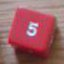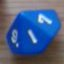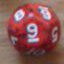How to manage pairs

## How to manage pairs

 Sat Oct 24 10:58:25 2015   by   Daniel Hi Torben, how are you?I'm returning in my dice mechanics studies since a long time...so I don't remember how to manage pairs.I'll try a dice pool that use only d6(one for each skill level), and count pairs like in a Lier's Dice(Pirate Dice) game.Example:one 5 < two 3s < two 4s < three 2s < four 1s ...and so on...Do you have a tip? Sat Oct 24 13:13:06 2015   by   Torben You can create a pair by using square brackets and a comma, such as `[3,4]`. You can get the components using `%1` and %2.  But there are limitations: You can not have a collection of pairs.  So the obvious solution of creating a collection of pairs of numbers and set sizes and then picking the pair with the largest set size does not work.  Besides, you can't compare pairs directly.So you need a function to handle this.  The following function takes as arguments a pool of d6s, a currently best set size, the number that goes with that and a counter that goes from 6 to 0.  The call uses an initial set size of 0, 0 as the number for that set and the counter at 6.  The pool is 5d6, but you can easily change that.  The function also works for other dice sizes than 6, just set the initial counter to the dice size.`function bestSet(pool,size,num,i) =  if i = 0 then [size,num]  else (s := count i = pool; \ count number of dice equal to i        if s > size then call bestSet(pool, s, i, i-1)        else call bestSet(pool, size, num, i-1))call bestSet(5d6, 0, 0 , 6)`